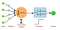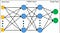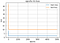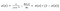# Target Prediction using Single-layer Perceptron and Multilayer Perceptron

This article is mainly written for beginners who want to start their journey into data science or willing to learn Neural Networks from scratch with python coding.

Objective:

• Basic Perceptron
• Multilayered Perceptron
• Dive into coding with a small dataset
• Optimizers
• Pipeline and App Deployment
• Conclusion

# Basic Perceptron

A perceptron is a neural network unit (an artificial neuron) that does certain computations to detect features or business intelligence in the input data.

Perceptron was introduced by Frank Rosenblatt in 1957. He proposed a Perceptron learning rule based on the original MCP neuron.

A Perceptron is an algorithm for the supervised learning of binary classifiers. This algorithm enables neurons to learn and processes elements in the training set one at a time.source: https://www.javatpoint.com/single-layer-perceptron-in-tensorflow

Single-layer Perceptrons can learn only linearly separable patterns. For classification we as Activation function as a threshold to predict class. And for Regression, we need not need the Activation function (Thresholding) or we can use a linear function to predict continuous value.

Input is typically a feature vector `x`multiplied by weights `w` and added to a bias `b`: `y = w * x + b`

where w denotes the vector of weights, x is the vector of inputs, b is the bias and φ is the non-linear activation function.

For Weight Updation or perceptron learn through backpropagation. we will see that in further section in detail.

# Multilayered Perceptron

Single-layer Perceptron is not able to figure out the nonlinearity or complexity of the data. So researchers developed the Multilayer perceptron using the idea of the single-layer perceptron.source: https://www.tutorialspoint.com/tensorflow/tensorflow_multi_layer_perceptron_learning.htm

Multiple Hidden layers are used to find the nonlinearity of the data. This instruction is also called a feed-forward network.

Multilayer perceptrons train on a set of input-output pairs and learn to model the correlation (or dependencies) between those inputs and outputs. Training involves adjusting the parameters, or the weights and biases, of the model to minimize error. Backpropagation is used to make those weigh and bias adjustments relative to the error.

Now, How the model learn from mistakes/errors? Let see the learning process:

Learning happens in two ways, Forward propagation and backward propagation

1. Forward Propagation: In the forward pass, the signal flow moves from the input layer through the hidden layers to the output layer, and the decision of the output layer is measured against the ground truth labels. And then will find error against ground truth and true label.
2. Back Propagation: Below is the steps mentioning how the back prop works.

In the above example, I have used SGD as an optimization algorithm for weight updates. But there are various optimization algorithm out there and I am going to use SGD, Momentum, and Adam for this article

# Optimization Algorithms:

Optimizers are algorithms or methods used to change the attributes of your neural network such as weights and learning rate to reduce the losses. For more information about optimizers check out this article

1. SGD

It’s a variant of Gradient Descent. It tries to update the model’s parameters more frequently. In this, the model parameters are updated after computation of loss on each training example. So, if the dataset contains 1000 rows SGD will update the model parameters 1000 times in one cycle of a dataset instead of one time as in Gradient Descent.

It means for every data point weights/parameter will update.

2. Momentum:

Momentum was invented for reducing high variance in SGD and softens the convergence. It accelerates the convergence towards the relevant direction and reduces the fluctuation to the irrelevant direction.

3. Adam :

Adaptive Moment Estimation(Adam) works with momentums of first and second order. The intuition behind the Adam is that we don’t want to roll so fast just because we can jump over the minimum, we want to decrease the velocity a little bit for a careful search. In addition to storing an exponentially decaying average of past squared gradients like AdaDelta, Adam also keeps an exponentially decaying average of past gradients

So, will sum up the history and introduction of Perceptron and multilayered perception including various optimization algorithms here. Now will move on to how this all works with an example (using small data set) and python coding from scratch.

# Dataset

For training single layer perception and Multilayer perception, I am using a small dataset. Let see how data look like,

We have 6 features and Target is the dependent variable that we have to predict. This is clearly a regression problem since the output variable is a continuous numerical value.

This above data set have their own equation which very domain-specific such as,

Where a,b,c,d,e,f are the unknown parameters.

To simplify the above equation will take log on both sides we get,

let,

we get,

Finally, we need to optimize the above equation and need to find optimized parameters like a,b,c,d,e,f.

# Single-layer Perceptron:

For this problem, I am using MSE as a loss function which can be defined for a single point as,

Now all equation has been defined except gradients,

Now we need to calculate gradients to update weights to minimize loss so that we get the optimal solutions. This we saw in backpropagation.

In the above figure, we are calculating the gradient for ‘a’. we will follow the arrow direction to calculate derivatives and we are going to use the chain rule.

Similarly, we are going to find derivatives for all parameters

Finally, we get the gradient matrix shown below,

Now it’s time to update weights,

Now we will implement all equations using python,

First, we will see forward propagation,

In the above code np.dot(X,w) will do matrix multiplication as we saw in the above equations

Now we will see backpropagation,

Now it’s time to parameter/weight update to reduce error/loss. First, we will use an SGD optimizer,Loss plot using SGD optimizer

We can clearly see from loss Vs. epoch plot that model is overfitting. That is train loss very low compare to test loss that means the model doing a good job of reducing training loss but fails to reduce test loss. Also, the difference between train and test loss is large that clearly indicating that the model is overfitting.

Now we will see what happen when we use the Momentum optimizer,

Here again same story repeats as we saw with the SGD optimizer.

Now we will see what happen when we use the Adam optimizer,

Here also a large gap between train and test loss.In general Single-layer perception not able to minimize the test loss. So we will see what happens if we use multilayer perception.

# Multilayer perception:

For this problem, we design Multilayer perception shown below,

Here I used only one hidden layer between input and output with the sigmoid activation function.

Weight matrix W1 (between the input layer and hidden layer) and W2(hidden layer and output layer) are,

Gradient Calculation for each weight/parameter is as follows,Sigmoid function and it's derivative

Finally, we get the gradient matrix as,

Until here we calculated derivatives of all parameters, Now it’s time to implement using python. First, we see the forward pass function,

All the equations with variable representation used in the code are the same we saw above theoretically.

Now we will see the backpropagation function,

First, we use SGD optimizer to update weights,

We can clearly see from the above plot MLP doing a good job both train and test loss are very close to each other so we can say the model is not overfitting.

Now we see what if we use Momentum optimizer,

We can see from the above plot SGD and Momentum works almost similarly.

Now we see what if we used Adam optimizer,

Adam reduces loss to a large extent compared to SGD and Momentum. So Adam performed well compared to SGD and Momentum optimizers.

In short multilayered Percptron works very well compared to single-layered perceptron.

# Pipeline and App Deployment

I have build end to end pipeline and deploy a web app using Stramlit and Heroku. The whole pipeline and deployment code is here. You can check out the whole code in my GitHub repo link mention below.

For Web App Demo, you can check out here

# Conclusion

As we see from loss Vs. epoch plots Clearly Multilayer Perceptron outperforms the single layer perception. As we have already seen the multilayer perception captures non-linearity from data. We haven’t used any libraries like TensorFlow and Keras which are developed to solve deep learning problems. But as you develop a more deep network (More than 1 hidden layer) then we must use these libraries as they are very well optimized.

Happy Learning !!!

## Nerd For Tech

From Confusion to Clarification

## Nerd For Tech

NFT is an Educational Media House. Our mission is to bring the invaluable knowledge and experiences of experts from all over the world to the novice. To know more about us, visit https://www.nerdfortech.org/. Don’t forget to check out Ask-NFT, a mentorship ecosystem we’ve started## Nerd For Tech

NFT is an Educational Media House. Our mission is to bring the invaluable knowledge and experiences of experts from all over the world to the novice. To know more about us, visit https://www.nerdfortech.org/. Don’t forget to check out Ask-NFT, a mentorship ecosystem we’ve started

## More From Medium

Medium is an open platform where 170 million readers come to find insightful and dynamic thinking. Here, expert and undiscovered voices alike dive into the heart of any topic and bring new ideas to the surface. Learn more

Follow the writers, publications, and topics that matter to you, and you’ll see them on your homepage and in your inbox. Explore

If you have a story to tell, knowledge to share, or a perspective to offer — welcome home. It’s easy and free to post your thinking on any topic. Write on Medium